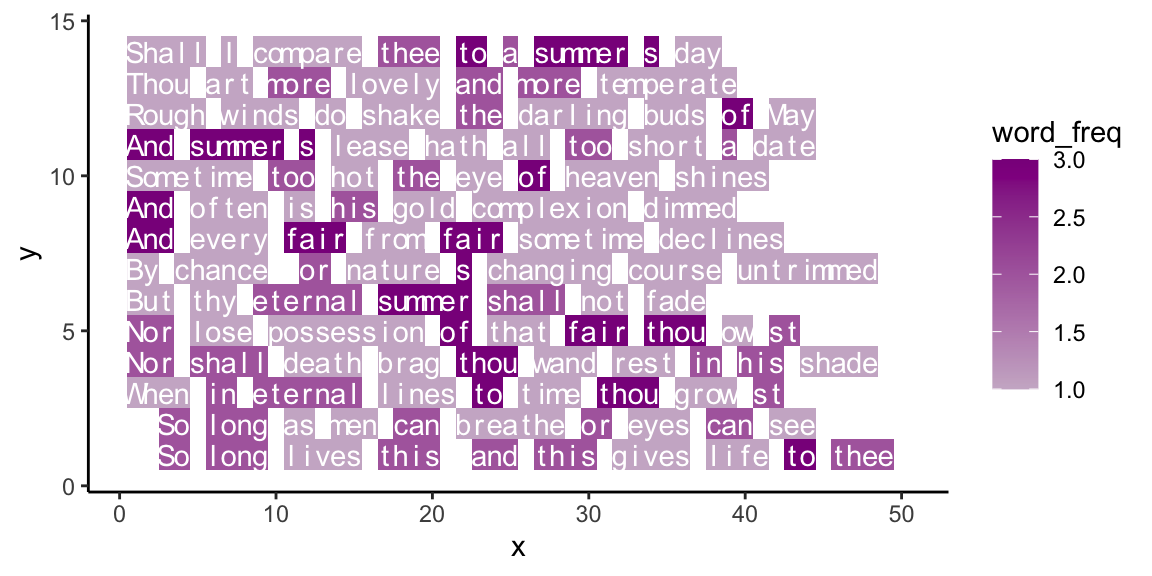## 20.5 ExercisesExercises on R pour l’art:

### 20.5.1 Visualizing mathematical puzzles

The so-called Collatz conjecture (see Wikipedia sounds like a simple math problem:

Starting with a positive integer $$n$$, compute the following numbers by:

• If the current number $$n$$ is even, compute the $$n+1$$. number as $$3n + 1$$;
• If the current number $$n$$ is odd, compute the $$n+1$$. number as $$n/2$$.

and repeat these rules to compute the next numbers.

The Collatz conjecture claims that regardless of its initial value $$n$$, the sequence will always reach 1.

Once a sequence reaches the value $$4$$, the sequence would get stuck in an infinite loop:
$$4$$ (even): $$4/2 = 2$$ (even): $$2/2 = 1$$ (odd): $$3+1 = 4$$, etc.
Hence, the condition of reaching the value of 1 stops the procedure, and will be met when reaching a value of 2 or 4.

1. Write an R function that returns the sequence for a given input value $$n$$.

2. Create a visualization that illustrates the sequences or some of their properties for a range of starting values.

3. Assume you wanted to verify the conjecture for a large number of initial values $$n$$ (e.g., $$n = 1, 2, ... 10^{10}$$). How could you make this more efficient than simply running your function for every candidate value?

Hint: The following video clip from the Veritasium channel explains the problem, provides some history, and contains lots of inspiring visualizations:

#### Solution

A corresponding function could use a while loop or use recursion.

• ad 1: A solution that uses a while loop (and considers some special cases):
Collatz <- function(n){

# Initialize:
seq <- n
target <- +1

# Catch some cases of special inputs:
if (n == 0){
stop("n = 0 would create an infinite loop.")
} else if (!ds4psy::is_wholenumber(n)){
stop("n is no integer, contrary to assumptions...")
} else if (n < 0){
warning("n < 0, contrary to assumptions. Checking for -1.")
target <- -1
}

# Loop:
while (n != target) {

# Apply rules:
if (n %% 2 == 0){  # x is even:
n <- n/2
} else if (n %% 2 == 1){ # x is odd:
n <- 3 * n + 1
}

seq <- c(seq, n)

} # while end.

return(seq)

}

# Check:
Collatz(1)
#>  1
Collatz(2)
#>  2 1
Collatz(3)
#>   3 10  5 16  8  4  2  1
Collatz(15)
#>    15  46  23  70  35 106  53 160  80  40  20  10   5  16   8   4   2   1
Collatz(27)
#>     27   82   41  124   62   31   94   47  142   71  214  107  322  161  484
#>   242  121  364  182   91  274  137  412  206  103  310  155  466  233  700
#>   350  175  526  263  790  395 1186  593 1780  890  445 1336  668  334  167
#>   502  251  754  377 1132  566  283  850  425 1276  638  319  958  479 1438
#>   719 2158 1079 3238 1619 4858 2429 7288 3644 1822  911 2734 1367 4102 2051
#>  [ reached getOption("max.print") -- omitted 37 entries ]

# Note that function works for negative integers:
Collatz(-4)
#>  -4 -2 -1
# but: Collatz(-5) gets stuck in a loop.
Collatz(-6)
#>  -6 -3 -8 -4 -2 -1

# Note errors for:
# Collatz(NA)
# Collatz(0)
# Collatz(1/2)

# Apply to range of values:
# sapply(X = 1:30, FUN = Collatz)
• As all numbers in the sequence follow the same rules, we can also create a recursive function (without considering cases of non-standard inputs):
Collatz_rec <- function(n){

if (n == 1) {

seq <- n  # stopping case

} else {

# Apply rules:
if (n %% 2 == 0){  # n is even:

n_new <- n/2

} else if (n %% 2 == 1){  # n is odd:

n_new <- 3 * n + 1

}

# Recursive step:
seq <- c(n, Collatz_rec(n_new))

}

return(seq)

}

# Check:
Collatz_rec(1)
#>  1
Collatz_rec(9)
#>    9 28 14  7 22 11 34 17 52 26 13 40 20 10  5 16  8  4  2  1

# Apply to range of values:
# sapply(X = 1:30, FUN = Collatz_rec)
• ad 3: There are many ways of writing more efficient functions. A key idea is that we do not need to (re-)check any number that occurs in a sequence that is known to conform to the conjecture. Thus, after checking a sequence and verifying that it reaches 1, we can add all its numbers to a store of “known numbers.” As soon as a sequence reaches any known number, we can stop checking it.

### 20.5.2 Visualizing word frequency

• Our example of plotting text in Section 20.3 used color to visualize the frequency of each character. Extend the example to visualize the frequency of words in a text.

#### Solution

Highlighting word frequency (using tb from above):

ggplot(tb, aes(x = x, y = y)) +
geom_tile(aes(fill = word_freq)) +
geom_text(aes(label = char), col = "white", fontface = 1) +
scale_fill_continuous(low = "thistle3", high = "darkmagenta",
# low = "red3", high = "darkred",
# low = "deepskyblue", high = "darkslateblue",
guide = "colorbar", na.value = "white") +
# coord_equal() +
theme_classic()Figure 20.6: Text with word frequency.

### 20.5.3 Creative freedom

• Create and solve your own exercise that involves some sort of creative expression in R.